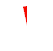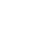Making Circular Health Bars/Progress Bars in GameMaker

We are going to go through two very basic scripts that can be used to draw the above charts. One script is to do the full circle bar (which is basically a pie chart) and the second script is for the ring progress bar. I have split it into two separate scripts so you can just add the ones you want to your game, also the pie chart runs a little more efficiently so where possible I recommend using that one. I will first go through the pie chart as this is a little easier and the ring bar is basically the same with a hole punched out of the middle.

draw_pie()This is how you call the script:

```draw_pie(x ,y ,health, 100, c_red, 20, 1)
```

This is the script you need to add:

```/// draw_pie(x ,y ,value, max, colour, radius, transparency)

if (argument2 > 0) { // no point even running if there is nothing to display (also stops /0
var i, len, tx, ty, val;

var numberofsections = 60 // there is no draw_get_circle_precision() else I would use that here
var sizeofsection = 360/numberofsections

val = (argument2/argument3) * numberofsections

if (val > 1) { // HTML5 version doesnt like triangle with only 2 sides

draw_set_colour(argument4);
draw_set_alpha(argument6);

draw_primitive_begin(pr_trianglefan);
draw_vertex(argument0, argument1);

for(i=0; i<=val; i++) {
len = (i*sizeofsection)+90; // the 90 here is the starting angle
tx = lengthdir_x(argument5, len);
ty = lengthdir_y(argument5, len);
draw_vertex(argument0+tx, argument1+ty);
}
draw_primitive_end();

}
draw_set_alpha(1);
}
```

How this works is by drawing a series of triangles that fan out to draw a circle, however I stop drawing them once we have reached the correct value.

I actually break the circle into 30 triangles as this seemed like a good balance between having a good looking circle and performing really well.

We use draw_primitive_begin(pr_trianglefan) to draw the triangles, what this does is once we have drawn the first 3 points every point after this will become its own triangle. This is why in the loop we only draw 1 point and GameMaker works out from the last triangle it drew where the other two points on this triangle are.

How do we find out where each of these new points should be? lengthdir_x() and lengthdir_y() are amazingly useful functions in GameMaker. You provide lengthdir_x() with a distance and a direction and using Pythagorean Theorem it will tell you the height of the triangle, likewise lengthdir_y() will tell you the width. These are really useful and you should learn how to use them.

draw_circular_bar()This is how you call the script:

```draw_circular_bar(x ,y ,health, 100, c_red, 20, 1, 6)
```

This is the script you need to add:

```/// draw_circular_bar(x ,y ,value, max, colour, radius, transparency, width)

if (argument2 > 0) { // no point even running if there is nothing to display (also stops /0
var i, len, tx, ty, val;

var numberofsections = 60 // there is no draw_get_circle_precision() else I would use that here
var sizeofsection = 360/numberofsections

val = (argument2/argument3) * numberofsections

if (val > 1) { // HTML5 version doesnt like triangle with only 2 sides

piesurface = surface_create(argument5*2,argument5*2)

draw_set_colour(argument4);
draw_set_alpha(argument6);

surface_set_target(piesurface)

draw_clear_alpha(c_blue,0.7)
draw_clear_alpha(c_black,0)

draw_primitive_begin(pr_trianglefan);
draw_vertex(argument5, argument5);

for(i=0; i<=val; i++) {
len = (i*sizeofsection)+90; // the 90 here is the starting angle
tx = lengthdir_x(argument5, len);
ty = lengthdir_y(argument5, len);
draw_vertex(argument5+tx, argument5+ty);
}

draw_primitive_end();

draw_set_alpha(1);

draw_set_blend_mode(bm_subtract)
draw_set_colour(c_black)
draw_circle(argument5-1, argument5-1,argument5-argument7,false)
draw_set_blend_mode(bm_normal)

surface_reset_target()

draw_surface(piesurface,argument0-argument5, argument1-argument5)

surface_free(piesurface)

}

}
```

As you can see most of this is the same as the first example just drawn onto a surface. If you haven’t used surfaces I have some guides on masking using surfaces and scratch cards that teach in more depth how to use surfaces in GameMaker. However they are basically an empty canvas you can draw onto in the same way you draw any sprite onto the screen and anything you draw there is saved, these surfaces can then themselves be drawn onto the screen with everything you have added onto them.

How the circular bar is working is by drawing the pie chart, however we draw it onto a surface using surface_set_target(), this allows us to further edit the pie chart after we have drawn it. We set the draw mode to mb_subtract using draw_set_blend_mode(bm_subtract) and this tells GameMaker that anything we draw from this point on shouldn’t be drawn as normal but should erase anything we draw. We use this to basically draw a hole out of the middle of the pie chart and this gives us our new ring chart.

We can then draw this radial chart onto the screen using draw_surface() and remove it from the memory using surface_free().

surface_exists():

I want to say here that it is really important to check to make sure a surface exists before you try and use them, surfaces can be volatile in GameMaker so always remember to check. After a lot of testing it doesn’t look like you have to in the above case because you have just created it in the same frame. You could add it if you were being safe or if your game depended on it.

HTML5:

To get it working in HTML5 you need to do some tinkering because you cant use a surface the way I am in a script on the HTML5 version, however if you were to move them into an object it would work great. The reason I didnt here is because scripts are just more accessible to everyone and easier to implement.